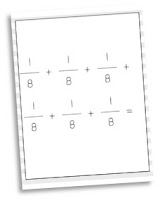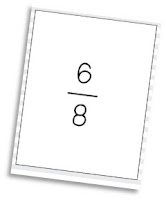# Multiplying Fractions by a Whole Number

Fraction concepts are taken up a level in 5th grade and many of the topics are very abstract and difficult for students to understand. Multiplying fractions by a whole number doesn’t need to be one of these difficult concepts! By using the CRA (Concrete-Representative-Abstract) framework your students will link what they already know about multiplication to the multiplication of fractions and will be successful in no time at all!

### Concrete Multiplying Fractions by a Whole Number

Before you begin with fractions, anchor your students back into what it means to multiply. Give them a pile of blocks and ask them to model something super simple such as “4×3” your 5th graders should quickly and easily be able to model this problem. Ask your students about what they built and how they know it represents the equation.

Go ahead and get out your pattern blocks. They are the PERFECT fraction representation. Let the hexagon represent a whole and allow your students to figure out which blocks represent 1/3 (rhombus), 1/2 (trapezoid) and 1/6 (triangles). Once your students are all set with their blocks, they are ready to begin! Don’t have fraction blocks handy? Fraction circles would work just as well!

Ask your students “If 4 groups of 3 blocks represented 4×3, how could we represent 4 x 1/3?” Allow students to use the rhombus pattern blocks to represent 4 groups of 1/3. The beauty of using blocks is that students can put these blocks back together to see both the improper fraction and mixed number that is created when fractions are multiplied together.

**This PICTURE is not a concrete model, but if you are creating this model out of fraction tiles, your students are working at the concrete level! **

### Representative Multiplying Fractions by a Whole NumberOnce your students are able to model 4 x 1/3 you want them to link this understanding to a representative model such as repeated addition. You may begin by asking your students to write a repeated addition equation to represent 4 x 3. This is easy for your students! Now, ask them to  use what they know about multiplication to write a repeated addition equation that represents 4 x 1/3.

### Abstract Multiplying Fractions by a Whole NumberThe concrete and representative steps of this activity allow your students to clearly understand what is going on when multiplying a fraction by a whole number. After your students have had a good deal of exposure at the concrete and representative level, give them a new equation such as 4 x 2/8 and ask your students what they *think* the product will be. You are looking for your students to make generalizations about their multiplication and fraction understandings and to be able to explain their thinking. After a student shares their thinking, ask all students to model with concrete materials or a repeated addition equation to confirm the product!

I have created a set of playing cards that includes multiplication equations, visual models, repeated addition and the resulting products. Your students can play so many traditional card games with this deck of cards – and I have included the instructions for 5 games to get you started! This resource is PERFECT for exploring the link between repeated addition and fraction multiplication. Click HERE to check it out!